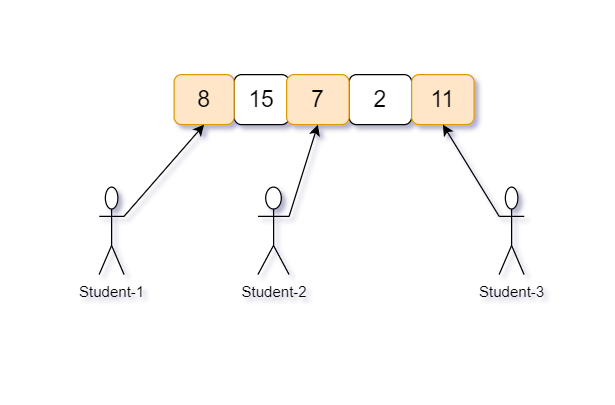New update is available. Click here to update.

# Chocolate Problem

Last Updated: 26 Nov, 2020
Difficulty: Moderate

## PROBLEM STATEMENT

#### Example :

``````Given 'N' : 5 (number of packets) and 'M' : 3 (number of students)
````````````And chocolates in each packet is : {8, 11, 7, 15, 2}

All possible way to distribute 5 packets of chocolates among 3 students are -

( 8,15, 7 ) difference of maximum-minimum is ‘15 - 7’ = ‘8’
( 8, 15, 2 ) difference of maximum-minimum is ‘15 - 2’ = ‘13’
( 8, 15, 11 ) difference of maximum-minimum is ‘15 - 8’ = ‘7’
( 8, 7, 2 ) difference of maximum-minimum is ‘8 - 2’ = ‘6’
( 8, 7, 11 ) difference of maximum-minimum is ‘11 - 7’ = ‘4’
( 8, 2, 11 ) difference of maximum-minimum is ‘11 - 2’ = ‘9’
( 15, 7, 2 ) difference of maximum-minimum is ‘15 - 2’ = 13’
( 15, 7, 11 ) difference of maximum-minimum is ‘15 - 7’ = ‘8’
( 15, 2, 11 ) difference of maximum-minimum is ‘15 - 2’ = ‘13’
( 7, 2, 11 ) difference of maximum-minimum is ‘11 - 2’ = ‘9’

Hence there are 10 possible ways to distribute ‘5’ packets of chocolate among the ‘3’ students and difference of combination (8, 7, 11) is ‘maximum - minimum’ = ‘11 - 7’ = ‘4’ is minimum in all of the above.
``````
##### Input format :
``````The first line of input contains an integer ‘T’ denoting the number of test cases.
The next ‘2*T’ lines represent the ‘T’ test cases.

The first line of each test case contains two space-separated integers ‘N’ denoting the number of packets of chocolate and ‘M’ denotes the number of students.

The second line of each test case contains ‘N’ space-separated integers denoting the number of chocolate in each of ‘N’ packets.
``````
##### Output Format :
``````For each test case, print the minimum difference of the chocolates contained in the packets distributed to the students.
``````
##### Note :
``````You don't need to print anything, it has already been taken care of. Just implement the given function.
``````
##### Constraints:
``````1 <= T <= 50
2 <= M <= N <= 10^4
1 <= CHOCOLATES[i] <= 10^9

Time Limit : 1 sec
``````